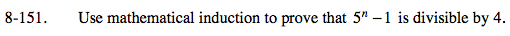### Home > PC > Chapter 8 > Lesson 8.2.6 > Problem8-151

8-151.51 − 1 = 4

Assume that 5k − 1 is divisible by 4, therefore 5k − 1 = 4A.

5k + 1 − 1 =
5(5)k − 1 =
5(5)k − 5 + 4 =
5(5k − 1) + 4 =
20A + 4 = 4(5A + 1)
So 5k + 1 − 1 is a multiple of 4.

Hence, by mathematical induction we have proven that 5n − 1 is divisible by 4 for all natural numbers.